# SSAT Elementary Level Math : How to find length of a line

## Example Questions

← Previous 1

### Example Question #1 : Lines

Which is true about a ray?

A ray is a line with a start point, but no end point.

A ray extends indefinitely in both directions.

A ray is always shaped like a circle.

A ray has three end points.

A ray is always shorter than a line segment.

A ray is a line with a start point, but no end point.

Explanation:

A ray always has one start point, and then continues indefinitely in the opposite direction. Line segments are different because they have a defined start and end point.

### Example Question #2 : How To Find Length Of A Line

Alex needs to buy a fence for her yard. She doesn't know how much she needs to surround the entire yard. What does she need to calculate to figure this out?

area of the yard

volume of the yard

height of the fence

perimeter of the yard

perimeter of the yard

Explanation:

Perimeter is the distance around a shape. She needs to know the perimeter of her yard to determine how much fence to buy.

### Example Question #1 : How To Find Length Of A Line

Line AC is 12 inches long. If Point B is located in the center of Line AC, how many inches long is Line BC?Explanation:

Since Point B is located in the middle of Line AC, it will break Line AC into two equal line segments - Line AB and Line BC. We should divide the original length of Line AC by 2 because we are breaking the original line in half.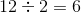### Example Question #2 : How To Find Length Of A Line

A line isinches long on a number line, if the starting point is, what is the end point on the number line?Explanation:

You must add the start point to the length of the line to find the end point of the line.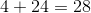### Example Question #4 : Lines

A pentagon has ____ sides.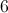Explanation:

A pentagon has five sides.

### Example Question #1 : Lines

Line AC is 24 inches long. If Point B is the midpoint of Line AC, how many inches long is Line BC?Explanation:

Point B is the midpoint that breaks Line AC into two line segments of equal length.  Therefore, to find the length of Line BC, divide the length of Line AC by 2: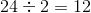### Example Question #3 : Lines

What is a line segment?

A line that has a start point but no end point.

A part of a line that connects two points.

A line that is always smaller than 10 centimeters.

A line that has no start or end points.

A line that is always greater than 10 centimeters.

A part of a line that connects two points.

Explanation:

A line segment is a part of a line that connects two points. It is different than a line and a ray because it has a defined start and end point

### Example Question #4 : Lines

Which statement is true regarding line segments?

A line segment is a "piece" of a line and has two endpoints.

A line segment has one endpoint, but continues indefinitely in one direction.

A line segment has three endpoints.

A line segment extends in both directions without endpoints.

A line segment is curved.

A line segment is a "piece" of a line and has two endpoints.

Explanation:

A line segment is a piece of a line connected by two endpoints.

### Example Question #9 : How To Find Length Of A Line

A line is connected from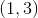to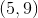.  What is the length of the line?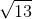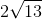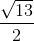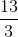Explanation:

Write the distance formula.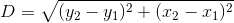Substitute the points and solve for the distance.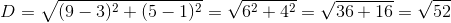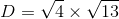### Example Question #8 : How To Find Length Of A Line

Find the length of a line which starts at the point (1,7) and goes till (9,7).Explanation:

To solve, simply realize the distance between these points will just be the difference of their x values since their y values stay the same.

These points are on a horizontal line.

Thus,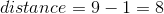← Previous 1